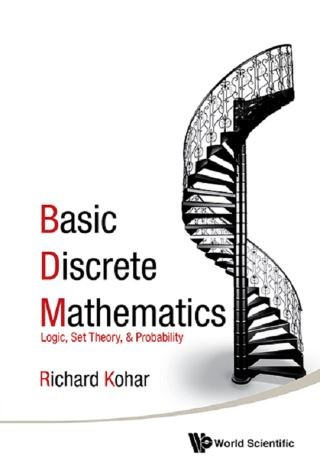## Basic Discrete Mathematics• Description
• Author
• Info
• Reviews

### Description

This lively introductory text exposes the student in the humanities to the world of discrete mathematics. A problem-solving based approach grounded in the ideas of George Pólya are at the heart of this book. Students learn to handle and solve new problems on their own. A straightforward, clear writing style and well-crafted examples with diagrams invite the students to develop into precise and critical thinkers. Particular attention has been given to the material that some students find challenging, such as proofs. This book illustrates how to spot invalid arguments, to enumerate possibilities, and to construct probabilities. It also presents case studies to students about the possible detrimental effects of ignoring these basic principles. The book is invaluable for a discrete and finite mathematics course at the freshman undergraduate level or for self-study since there are full solutions to the exercises in an appendix.

Contents:
• Introduction to Logic
• Proofs and Arguments
• Sets and Set Operations
• Infinity
• Elements of Combinatorics
• Sequences and Series
• The Binomial Theorem
• Introduction to Probability
• Random Variables
• Probability Distributions

Key Features:
• One of the first books written on discrete mathematics for the humanities audience
• Litte mathematical knowledge is assumed. Great for humanities, liberal arts, and motivated high-school students
• Uses Pólya's approach to problem solving: understand the problem, devise a plan, carry out the plan, and look back
• Full solutions to all exercises included
• Clear layout of text with essential figures